# Numbers and Operations

## Numeracy and confidence are at the heart of math success

Confidence and competency in basic arithmetic lies at the heart of success in math. We believe every kid is capable of mastering arithmetic. However, it is critical that both a good conceptual understanding and a fair degree of proficiency and confidence is established in each skill area before moving on to harder topics.  In math, skills build on each other.

## Numbers and operations activities

Numbers and operations starts with simple number recognition and counting, moves to larger numbers and place value concepts and then continues through the 4 operations to fractions, decimals, percents and concepts around ratios and factoring.

• number recognition
• numbers and counting
• text and numbers
• ordinal numbers
• odd and even numbers
• the number line
• comparing and ordering numbers
• counting by grouping
• place value
• building number sentences
• multiplication and division
• rounding and estimating
• fractions, equivalent fractions, improper fractions
• decimals, percents and ratios
• factors, prime factors and multiples
• least common denominator
• powers of 10 and exponents

## Numbers and operations worksheets

Our free numbers and operations worksheets are organized in the following topic areas:

Learning Numbers

Recognizing and printing numbers, ordinal numbers and odd / even numbers.

Counting

Counting objects, sequential counting, skip counting & counting backwards.

Comparing Numbers

Concepts of more, less, most, and least, comparing groups of objects, comparing numbers & ordering numbers.

Place Value

Base 10 blocks, tens and ones, composing and decomposing numbers, normal and expanded form, place values of up to 6 digit numbers.

Rounding

Rounding numbers of varying sizes to the nearest 10, 100 or other power of 10.

From counting a few objects using pictures up to adding multiple large numbers in columns with regrouping.

Subtraction

From subtracting by "taking away" objects using pictures to subtracting large numbers in columns with regrouping.

Multiplication

From basic multiplication facts to multiplying multi-digit whole numbers in columns.

Division

From basic division facts to long division of whole numbers with/without remainders.

Order of operations (PEMDAS)

Order of operations focused on the 4 operations and use of parenthesis.

Roman numerals

Fractions

From the concepts of "equal parts" through adding, subtracting, multiplying and dividing proper and improper fractions and mixed numbers.

Decimals

From converting simple fractions to/from decimals to the long division of multi-digit decimals.

Factoring & prime factors

Factors, prime factors, greatest common factors (GCFs) and least common multiples (LCMs).

Exponents

Exponent notation including whole number, fractional and decimal bases, negative exponents, equations with exponents.

Proportions

Whole number and decimal proportions and proportion word problems.

Percents

Common calculations involving percents.

Integers

Addition, subtraction, multiplication and division of integers (whole numbers which can be negative or positive).

## Numbers and operations workbooks

Our numbers and operations workbooks are listed below.  Target grade levels are shown on each book cover.  Full descriptions including a table of contents and sample pages are provided in our bookstore.Numbers and Counting to 10 Introduces students to numbers up to 10 including printing numbers, patterns, words for numbers, counting and comparing numbers.Numbers and Counting to 100 Introduces students to numbers from 11 - 100, including concepts such as "tens and ones", "same and different", sorting & sets.Add and Subtract Introduces addition and subtraction, focusing on adding and subtracting up to 10 objects.  Includes number bonds and simple equations.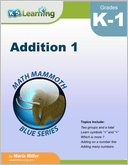Addition 1 The concept of addition and addition facts within 0-10. Introduces number lines and symbols for addition.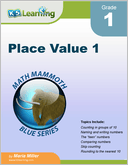Place Value 1 Covers 2-digit numbers, with emphasis on "tens and ones". Includes skip counting, rounding to the nearest 10 and adding / subtracting tens.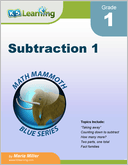Subtraction 1 Concept of subtraction and subtraction facts within 0-10.  Includes "taking away", counting down and fact families.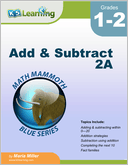Add and Subtract 2A Adding and subtracting within 0-20, adding doubles, subtraction strategies, completing the next ten, fact families and single digit subtraction facts.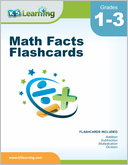Math Facts Flashcards Flashcards for addition, subtraction, multiplication and division facts, 0-12.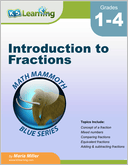Introduction to Fractions Introducing fractions, mostly for grades 3-4. Concept of a fraction, mixed numbers, comparing fractions, equivalent fractions, adding and subtracting fractions and fractions multiplied by a whole number.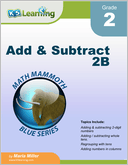Add and Subtract 2B Addition and subtraction within 0-100, both mentally and in columns, especially concentrating on regrouping ("carrying" in addition and "borrowing" in subtraction).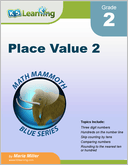Place Value 2 Three digit numbers; place values of ones, tens and hundreds.  Number lines, skip counting by tens, rounding to the nearest ten or hundred, bar graphs and picture graphs.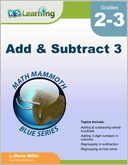Add & Subtract 3 Adding and subtracting 3-digit numbers, mentally and in columns, within 1-1,000. Includes regrouping tens as hundreds and regrouping across zeros. Also ordinal numbers, roman numerals and order of operations.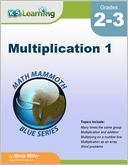Multiplication 1 Introducing the concept of multiplication and the order of operations.  The second part of the book focuses on the memorization of the multiplication tables.  Includes word problems.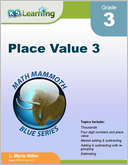Place Value 3 Four digit numbers; place values of ones, tens, hundreds and thousands.  Topics include comparing numbers, adding and subtracting with regrouping, rounding, estimating and word problems.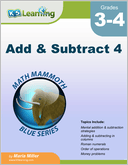Add and Subtract 4 Mental addition and subtraction strategies; adding and subtracting in columns and order of operations.  Also Roman numerals, money problems and bar and line graphs.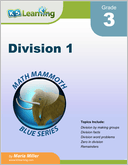Division 1 Introducing division, in particular single digit division, remainders and divisibility.  Division by making groups, division facts and division word problems.  Remainders and divisibility rules.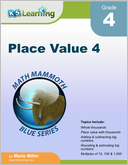Place Value 4 Numbers and place value in the thousands, ten thousands, hundred thousands and millions.  Adding, subtracting, rounding and estimating big numbers.  Multiples of 10, 100 and 1,000.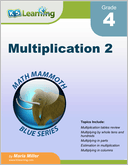Multiplication 2 Multi-digit multiplication including multiplying in parts, multiplying in columns and order of operations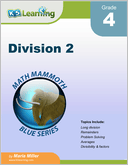Division 2 A continuation of division topics, including long division, averaging and factors.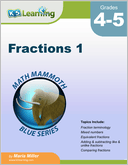Fractions 1 Focus on the addition and subtraction of fractions with like and unlike denominators.  Also mixed numbers, equivalent fractions and comparing fractions.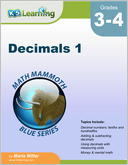Decimals 1 Introduction to decimals (tenths and hundredths). Adding and subtracting decimals mentally and in columns.  Decimals and money.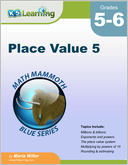Place Value 5 Numbers up to the trillions, rounding huge numbers and using a calculator.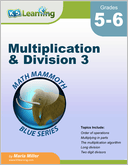Multiplication and Division 3 Multiplication and division topics including order of operations, multiplying in parts, long division, divisibility rules, factors and prime factors, greatest common factor, least common multiple and ratios.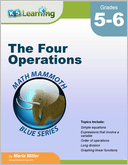The Four Operations Topics related to the four operations and algebra including balance problems and equations, expressions involving a variable, order of operations, the multiplication algorithm, long division, powers and exponents and the coordinate grid.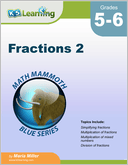Fractions 2 Simplifying fractions and the multiplication and division of fractions and mixed numbers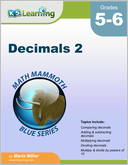Decimals 2 Adding, subtracting, multiplying and dividing decimals.  Multiplying and dividing by powers of 10. Rounding and estimating.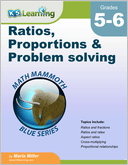Ratios, Proportions & Problem Solving Ratios and fractions, ratios and rates, cross multiplying, proportional relationships, aspect ratios, scaling figures, bar models and significant digits.